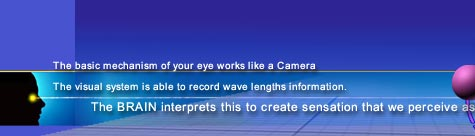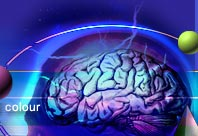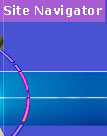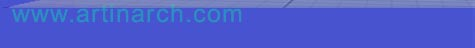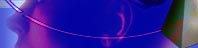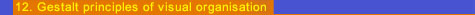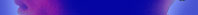The basic concept of' figure' and 'ground' is an outcome of Gestalt psychologists, Max Wertheimer (1880 -- 1943), Wolfgang Koher (1887 -- 1967) and Kurt Koffka (1886 -- 1941). These psychologists advocated that when an individual is confronted by any ( abstract or symbolic ) visual image, we seem to separate a dominant shape (a 'figure' with definite contours) from the background. The famous ambiguous figure devised by the Danish psychologist Edgar Rabin (figure 12) is the most appropriate example to explain the figure ground relationship. In addition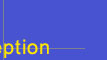workshop theory slide galleryto introducing the terms 'figure' and 'ground' Gestalt psychologists outlined several fundamental irreversible principles of perception and organisation.(figure 12) 12.01 - PROXIMITY : (figure 13, 14, 15) The columns or rows dominate our focus. The features which are close to each other are associated. In figure 15 we are more unlikely to associate the lines which are close to each other than those which are further apart.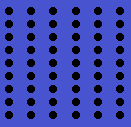(figure 13) (figure 15) (figure 14) 12.02 - SIMILARITY : (figure 16 & 17) Features which look similar are associated. The circles and squares are associated naturally and we do tend to see alternating columns of circles and squares. Without the two different recurrent features we would perceive the figure 17 as either rows or columns. (figure 16)(figure 17) 12.03 - CONTINUITY : (figure 18) The lines based on smooth continuity are preferred over abrupt changes of direction. In this figure we are more likely to identify lines a -- b & c -- d. crossing each other than to identify a -- d and c - b or a - c & d - b as lines.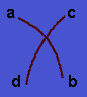(figure 18) 12.04 - CLOSURE : (figure 19 & 20) In this figure we tend to see three broken rectangles and a lonely box bracket ] on the far left rather than three girder profiles and a lonely box bracket ] on right. In this case the principle of closure cuts across the principle of proximity. If we now remove the horizontal lines we then return to the image used earlier to illustrate proximity.(figure 19)(figure 20) 12.05 - SMALLNESS : (figure 21 & 22) Smaller areas tend to be seen as figures against larger background. In these figures we are likely to see a black cross rather than a white cross. (figure 21)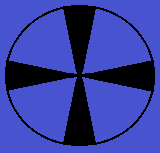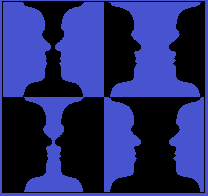(figure 22) 12.06 - SYMMETRY : (figure 23) The principle of symmetry is that, the symmetrical areas tend to be seen as figures against the asymmetrical background.(figure 23) 12.07 - SURROUNDNESS : (figure 24) According to this principle areas which can be seen as surrounded by others tend to be perceived as figures. In this figure the word is initially confused by resuming the black area as a ground. Once it assumes the background as surrounding area then you can discover the world 'Tie'.(figure 24) « back to topics

For more details contact - Ar. Shirish Sukhatme
| Workshop | Theory | Slide Gallery |
| Home | About the author | Endorsements | Query | Contact | Feedback |